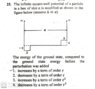# Infinitesimal Perturbation in a potential well

• Baibhab Bose

#### Baibhab Bose

Homework Statement
In an infinite potential well, two small constant perturbations \epsilon are added in the two opposite corners of the well in an infinitesimal extent (\delta). In what order of \epsilon the correction to the ground state energy would be? See the attached file.
Relevant Equations
<psi|Hp|psi>=E1
If I calculate ## <\psi^0|\epsilon|\psi^0>## and ## <\psi^0|-\epsilon|\psi^0>## separately and then add, the correction seems to be 0 since ##\epsilon## is a constant perturbation term.
SO how should I approach this? And how the Δ is relevant in this calculation?

#### Attachments

•NETQ1.jpg
59.2 KB · Views: 405

Yes, the first-order correction is zero. Are you familiar with how to calculate the second-order correction to the energy?

If I calculate ## <\psi^0|\epsilon|\psi^0>## and ## <\psi^0|-\epsilon|\psi^0>## separately and then add, the correction seems to be 0 since ##\epsilon## is a constant perturbation term.
Careful with the notation. ## <\psi^0|\epsilon|\psi^0>## and ## <\psi^0|-\epsilon|\psi^0>## are not what you need to calculate for the first-order correction.

Rather, the expression you need to consider is ##<\psi^0|V(x)|\psi^0>##, where ##V(x)## is the perturbation:

##V(x) = -\epsilon## for ##0<x<\Delta##
##V(x) = + \epsilon## for ##a-\Delta < x < a##
##V(x) = 0##, otherwise.

(Here I assumed the origin x = 0 is at the left end of the well.)

For example, your expression ## <\psi^0|-\epsilon|\psi^0>## does not take into account that the perturbation ##-\epsilon## is only over the range ##0<x<\Delta##.

•Baibhab Bose
Yes, I know how 2nd order is calculated. $$E^2_0=\sum_{n=/m} \frac{(<\psi_n|V|\psi_m>)^2}{E^0_n-E^0_m}$$
But I can't seem to practically calculate the correction in this case. Assuming ##\psi_n##s are ##\sqrt {\frac{2}{a}}sin(\frac{n\pi x}{a})## , what are the values of n's I'd have to limit to?

$$E^2_0=\sum_{n=/m} \frac{(<\psi_n|V|\psi_m>)^2}{E^0_n-E^0_m}$$
The right-hand side is not quite correct. There is nothing on your right-hand side that indicates that you are getting the second-order correction to the ground state. Also, be careful that you have the terms in the denominator in the correct order.

You will not have to work out the values of the matrix elements in order to answer the question. You will just need to consider whether or not the right-hand side overall is positive, negative, or zero.

Oh yes, so the correct form would be ## E_0^2=\sum_{n\neq m} \frac {(<\psi_m^0|V|\psi_0^0>)^2}{E^0_0-E^0_m}## for the ground state.
So in this question we just need the order of ##\epsilon## which, by speculation, would be ##\epsilon^2## since the 2nd order correction depends on square of the perturbation term!
But how do we ensure its sign?

Oh yes, so the correct form would be ## E_0^2=\sum_{n\neq m} \frac {(<\psi_m^0|V|\psi_0^0>)^2}{E^0_0-E^0_m}## for the ground state.
This still needs a little fixing up. Your summation notation indicates that ##n## is the summation index, but the index ##n## does not appear in the expression following the summation symbol. Instead, it looks like maybe your expression is for ##m## as the summation index. Anyway, for whatever symbol you take to be the summation index, what particular value of this index is to be excluded in the summation?

So in this question we just need the order of ##\epsilon## which, by speculation, would be ##\epsilon^2## since the 2nd order correction depends on square of the perturbation term!
But how do we ensure its sign?
How does the value of ##E^0_0## compare to any other ##E^0_m##?

This still needs a little fixing up. Your summation notation indicates that ##n## is the summation index, but the index ##n## does not appear in the expression following the summation symbol. Instead, it looks like maybe your expression is for ##m## as the summation index. Anyway, for whatever symbol you take to be the summation index, what particular value of this index is to be excluded in the summation?

How does the value of ##E^0_0## compare to any other ##E^0_m##?
## E_0^2=\sum_{m\neq 0} \frac{(<\psi^0_m|V|\psi^0_0>)^2}{E_0^0-E^0_m}##
Now,
##E_0^0##= unperturbed Ground state Wavefunction's Energy.
##E^0_m##=Unperturbed energy eigenvalue of the higher state wavefunctions, since m index represents states higher than the ground state, ##E_0^0-E^0_m## should be negative, right?